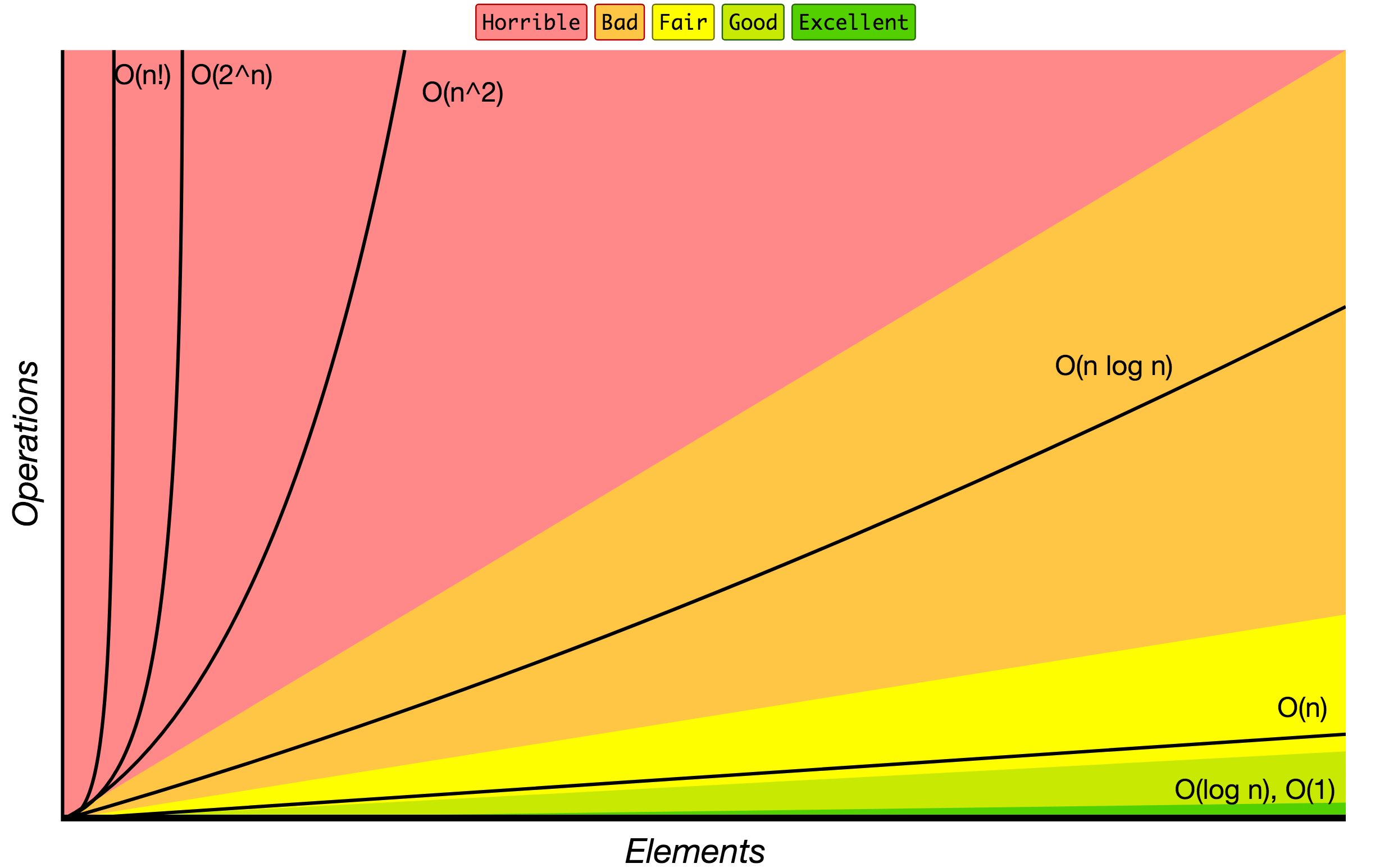# 基本算法复杂度统计

### Big O Notation

Big O notation is used to classify algorithms according to how their running time or space requirements grow as the input size grows.
On the chart below you may find most common orders of growth of algorithms specified in Big O notation.Source: Big O Cheat Sheet.

Below is the list of some of the most used Big O notations and their performance comparisons against different sizes of the input data.

Big O Notation Computations for 10 elements Computations for 100 elements Computations for 1000 elements
O(1) 1 1 1
O(log N) 3 6 9
O(N) 10 100 1000
O(N log N) 30 600 9000
O(N^2) 100 10000 1000000
O(2^N) 1024 1.26e+29 1.07e+301
O(N!) 3628800 9.3e+157 4.02e+2567

### Data Structure Operations Complexity

Data Structure Access Search Insertion Deletion Comments
Array 1 n n n
Stack n n 1 1
Queue n n 1 1
Linked List n n 1 n
Hash Table - n n n In case of perfect hash function costs would be O(1)
Binary Search Tree n n n n In case of balanced tree costs would be O(log(n))
B-Tree log(n) log(n) log(n) log(n)
Red-Black Tree log(n) log(n) log(n) log(n)
AVL Tree log(n) log(n) log(n) log(n)
Bloom Filter - 1 1 - False positives are possible while searching

### Array Sorting Algorithms Complexity

Name Best Average Worst Memory Stable Comments
Bubble sort n n2 n2 1 Yes
Insertion sort n n2 n2 1 Yes
Selection sort n2 n2 n2 1 No
Heap sort n log(n) n log(n) n log(n) 1 No
Merge sort n log(n) n log(n) n log(n) n Yes
Quick sort n log(n) n log(n) n2 log(n) No Quicksort is usually done in-place with O(log(n)) stack space
Shell sort n log(n) depends on gap sequence n (log(n))2 1 No
Counting sort n + r n + r n + r n + r Yes r - biggest number in array
Radix sort n * k n * k n * k n + k Yes k - length of longest key